Theory and Modern Applications

# Positive solution of singular BVPs for a system of dynamic equations on time scales

## Abstract

This paper is devoted to derivation of some necessary and sufficient conditions for the existence of positive solutions to a singular second-order system of dynamic equations with Dirichlet boundary conditions. The results are obtained by employing fixed-point theorems and the method of lower and upper solutions.

MSC:34B16, 34K10, 39A13.

## 1 Introduction

The main purpose of this paper is to establish existence results for the second-order Dirichlet system

$\left(P\right)\phantom{\rule{1em}{0ex}}\left\{\begin{array}{c}{x}_{i}^{\mathrm{\Delta }\mathrm{\Delta }}={f}_{i}\left(t,{x}^{\sigma }\left(t\right)\right),\phantom{\rule{1em}{0ex}}t\in {\left({J}^{\kappa }\right)}^{o},\hfill \\ {x}_{i}\left(a\right)={A}_{i},\phantom{\rule{2em}{0ex}}{x}_{i}\left({\sigma }^{2}\left(b\right)\right)={B}_{i},\phantom{\rule{1em}{0ex}}i=1,2,\dots ,n\hfill \end{array}$

with $f=\left({f}_{1},\dots ,{f}_{n}\right)$, $i=1,2,\dots ,n$, where ${f}_{i}:{\left({J}^{\kappa }\right)}^{o}×\mathcal{A}\to \mathbb{R}$, $\mathcal{A}\subset {\mathbb{R}}^{n}$ and J is a time scale interval. The nonlinearity ${f}_{i}\left(t,x\right)$ may be singular at ${x}_{i}$, $i=1,\dots ,n$ and/or t.

Stefan Hilger  introduced the notion of time scale in 1988 in order to unify the theory of continuous and discrete calculus. The time scales approach not only unifies differential and difference equations, but also solves some other problems such as a mix of stop-start and continuous behaviors [2, 3] powerfully. Nowadays the theory on time scales has been widely applied to several scientific fields such as biology, heat transfer, stock market, wound healing and epidemic models.

Under the general form of problem (P), it included the Emden-Fowler equation which arises, for example, in astrophysics in relation to the stellar structure (gaseous dynamics). In this case, the fundamental problem is to investigate the equilibrium configuration of the mass of spherical clouds of gas. It also arises in gas dynamics and fluid mechanics. The solutions of physical interest in this context are bounded non-oscillatory and possess a positive zero. It is also encountered in the relativistic mechanics and nuclear physics; and in chemically reacting systems: in the theory of diffusion and reaction this equation appears as governing the concentration u of a substance which disappears by an isothermal reaction at each point of a slab of catalyst. We refer to Wong  for a general historical overview of this equation.

Many works on this system have been written in the continuous case. We can cite, among others, [5, 6] or  for $n=1$ or  for $n=2$. On the discrete case, we find the book  which studies the oscillation properties of the solutions of different difference equations. For the specific problem ${u}^{\mathrm{\Delta }\mathrm{\Delta }}\left(t\right)+p\left(t\right){u}^{\gamma }\left(\sigma \left(t\right)\right)=0$, where $p\ge 0$ and γ is the quotient of odd positive numbers, oscillation properties were also studied in .

Regarding time scales, some results on the existence and uniqueness of classical solutions or solutions in the sense of distribution for $n=1$ can be found in the articles  and . Considering classical solutions, oscillation properties have also been studied in works such as  (with delay) or .

In the present paper, we present some results on time scales considering classical solutions which generalize the ones from the continuous case. The remainder of the paper is organized as follows. In Section 2, we state some existence results supposing the existence of a pair of lower and upper solutions and employing the Schauder fixed-point theorem. In Section 3, we shall give a necessary and sufficient condition for the existence of positive solutions of singular boundary value problem (P) by constructing a lower solution.

## 2 Lower and upper solutions method

Let $\mathbb{T}$ be an arbitrary time scale. We assume that $\mathbb{T}$ has the topology that it inherits from the standard topology on . See  for general theory on time scales.

Let $a,b\in \mathbb{T}$ be such that $a<\rho \left(b\right)$. If a is a right-dense point, we consider $J={\left(a,{\sigma }^{2}\left(b\right)\right]}_{\mathbb{T}}$, ${J}^{\kappa }={\left(a,\sigma \left(b\right)\right]}_{\mathbb{T}}$ and ${\left({J}^{\kappa }\right)}^{o}={\left(a,\sigma \left(b\right)\right)}_{\mathbb{T}}$. In the other case, $J={\left[a,{\sigma }^{2}\left(b\right)\right]}_{\mathbb{T}}$, ${J}^{\kappa }={\left[a,\sigma \left(b\right)\right]}_{\mathbb{T}}$ and ${\left({J}^{\kappa }\right)}^{o}={\left[a,\sigma \left(b\right)\right)}_{\mathbb{T}}$.

The problem we will consider in this section is

$\left(P\right)\phantom{\rule{1em}{0ex}}\left\{\begin{array}{c}{x}_{i}^{\mathrm{\Delta }\mathrm{\Delta }}={f}_{i}\left(t,{x}^{\sigma }\left(t\right)\right),\phantom{\rule{1em}{0ex}}t\in {\left({J}^{\kappa }\right)}^{o},\hfill \\ {x}_{i}\left(a\right)={A}_{i},\phantom{\rule{2em}{0ex}}{x}_{i}\left({\sigma }^{2}\left(b\right)\right)={B}_{i},\phantom{\rule{1em}{0ex}}i=1,2,\dots ,n.\hfill \end{array}$

with $f=\left({f}_{1},\dots ,{f}_{n}\right)$, $i=1,2,\dots ,n$, where ${f}_{i}:{\left({J}^{\kappa }\right)}^{o}×\mathcal{A}\to \mathbb{R}$, $\mathcal{A}\subset {\mathbb{R}}^{n}$.

We say that f verifies the hypothesis (H1) if for every $i=1,2,\dots ,n$, the following conditions are satisfied:

1. (i)

For every $x\in \mathcal{A}$, ${f}_{i}\left(\cdot ,x\right)\in {C}_{rd}\left({\left({J}^{\kappa }\right)}^{o}\right)$,

2. (ii)

${f}_{i}\left(t,\cdot \right)$ is continuous on $\mathcal{A}$ uniformly in $t\in {\left({J}^{\kappa }\right)}^{o}$.

For convenience, we denote

$E=\left\{g\in {C}_{rd}\left({\left({J}^{\kappa }\right)}^{o},{\mathbb{R}}^{+}\right):{\int }_{a}^{\sigma \left(b\right)}\left(\sigma \left(s\right)-a\right)\left({\sigma }^{2}\left(b\right)-s\right)g\left(s\right)\mathrm{\Delta }s<+\mathrm{\infty }\right\}.$

We say that f satisfies the condition (H2) on $\mathcal{B}\subset {\left({J}^{\kappa }\right)}^{o}×\mathcal{A}$ if for $i=1,2,\dots ,n$ there exists a function ${h}_{i}\in E$ such that

(H2) $|{f}_{i}\left(t,x\right)|\le {h}_{i}\left(t\right)$, $\mathrm{\forall }\left(t,x\right)\in \mathcal{B}$.

Definition 2.1 A solution of (P) is a function $x=\left({x}_{1},\dots ,{x}_{n}\right)$, with ${x}_{i}\in {C}_{rd}^{2}\left({\left(a,b\right)}_{\mathbb{T}}\right)$ for all $i=1,\dots ,n$ such that $x\left(t\right)\in \mathcal{A}$ for all $t\in {\left[a,{\sigma }^{2}\left(b\right)\right]}_{\mathbb{T}}$, which satisfies (P) for each $t\in {\left({J}^{\kappa }\right)}^{o}$ and $i=1,\dots ,n$, where

Definition 2.2 We say that $\alpha =\left({\alpha }_{1},\dots ,{\alpha }_{n}\right)$, with ${\alpha }_{i}\in {C}_{rd}^{2}\left({\left(a,b\right)}_{\mathbb{T}}\right)$, is a lower solution of (P) if $\alpha \left(t\right)\in \mathcal{A}$ for all $t\in {\left[a,{\sigma }^{2}\left(b\right)\right]}_{\mathbb{T}}$ and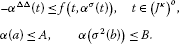An upper solution $\beta =\left({\beta }_{1},\dots ,{\beta }_{n}\right)$ of (P) is defined similarly by reversing the previous inequalities.

We have the following result.

Theorem 2.1 Let α and β be, respectively, a lower and upper solution for problem (P) such that $\alpha \le \beta$ on ${\left[a,{\sigma }^{2}\left(b\right)\right]}_{\mathbb{T}}$. If f satisfies (H1) and the condition (H2) on

${\mathcal{D}}_{\alpha }^{\beta }=\left\{\left(t,x\right)\in {\left({J}^{\kappa }\right)}^{o}×\mathbb{R}:{\alpha }^{\sigma }\left(t\right)\le x\le {\beta }^{\sigma }\left(t\right)\right\},$

and

(H3) For $t\in {\left[a,b\right]}_{T}$ and $x\in \mathcal{A}:\alpha \left(t\right)\le x\le \beta \left(t\right)⇒$

${f}_{i}\left(t,\alpha \left(t\right)\right)\le {f}_{i}\left(t,x\right)\le {f}_{i}\left(t,\beta \left(t\right)\right),$

for all $i=1,\dots ,n$.

Then problem (P) has at least one solution x such that $\alpha \le x\le \beta$ on ${\left[a,{\sigma }^{2}\left(b\right)\right]}_{\mathbb{T}}$.

Proof We consider the following modified problem:

$\left({P}_{m}\right)\phantom{\rule{1em}{0ex}}\left\{\begin{array}{c}{x}_{i}^{\mathrm{\Delta }\mathrm{\Delta }}\left(t\right)=-{f}_{i}^{\ast }\left(t,{x}^{\sigma }\left(t\right)\right),\phantom{\rule{1em}{0ex}}t\in {\left({J}^{\kappa }\right)}^{o},\hfill \\ {x}_{i}\left(a\right)={A}_{i},\phantom{\rule{2em}{0ex}}{x}_{i}\left({\sigma }^{2}\left(b\right)\right)={B}_{i},\phantom{\rule{1em}{0ex}}i=1,2,\dots ,n\hfill \end{array}$

with

${f}_{i}^{\ast }\left(t,x\right)={f}_{i}\left(t,{d}_{i}\left(t,x\right)\right)+\frac{{d}_{i}\left(t,x\right)-{x}_{i}}{1+|{d}_{i}\left(t,x\right)-{x}_{i}|},$

where $d=\left({d}_{1},\dots ,{d}_{n}\right)$ and ${d}_{i}:{\left[a,{\sigma }^{2}\left(b\right)\right]}_{\mathbb{T}}×\mathcal{A}\to \mathbb{R}$ is defined

We can prove that ${d}_{i}\left(t,\cdot \right)$ is continuous on $\mathcal{A}$ uniformly in t and ${d}_{i}\left(\cdot ,x\right)\in {C}_{rd}\left({\left[a,{\sigma }^{2}\left(b\right)\right]}_{\mathbb{T}}\right)$ for every $x\in \mathcal{A}$. Hence, the function $p:{\mathcal{D}}_{\alpha }^{\beta }\to {\mathcal{D}}_{\alpha }^{\beta }$, $p\left(t,x\right)=\left(t,d\left(t,x\right)\right)$ verified for each $x\in \mathcal{A}$, $p\left(\cdot ,x\right)\in {C}_{rd}\left({\left({J}^{\kappa }\right)}^{o}\right)$.

Due to the hypothesis, it is easy to see that (H1) is satisfied and that there exist ${h}_{i}^{\ast }\in E$ such that (H2) holds for the function ${f}^{\ast }$.

Note that if u is a solution of (${P}_{m}$) such that $\alpha \le u\le \beta$ on ${\left[a,{\sigma }^{2}\left(b\right)\right]}_{\mathbb{T}}$, then u is a solution of (P).

To show that any solution u of (${P}_{m}$) is between α and β, suppose that there exists $i=1,\dots ,n$ and ${t}^{\ast }\in {\left[a,{\sigma }^{2}\left(b\right)\right]}_{\mathbb{T}}$ such that ${v}_{i}\left({t}^{\ast }\right)={\alpha }_{i}\left(t\right)-{u}_{i}\left(t\right)>0$. As ${v}_{i}\left(a\right)\le 0$ and ${v}_{i}\left({\sigma }^{2}\left(b\right)\right)\le 0$, then there exists ${t}_{0}\in {\left(a,{\sigma }^{2}\left(b\right)\right)}_{\mathbb{T}}$ with

${v}_{i}\left({t}_{0}\right)=max\left\{{v}_{i}\left(t\right),t\in {\left[a,{\sigma }^{2}\left(b\right)\right]}_{\mathbb{T}}\right\}>0,$

and ${v}_{i}\left(t\right)<{v}_{i}\left({t}_{0}\right)$ for $t\in {\left({t}_{0},{\sigma }^{2}\left(b\right)\right]}_{\mathbb{T}}$. The point ${t}_{0}$ is not simultaneously left-dense and right-scattered (see Theorem 2.1 in ). This implies that $\left(\sigma \circ \rho \right)\left({t}_{0}\right)={t}_{0}$, and we have that ${v}_{i}^{\mathrm{\Delta }\mathrm{\Delta }}\left(\rho \left({t}_{0}\right)\right)\le 0$ (see ), so given that ${\alpha }^{\sigma }\left(t\right)\le d\left(t,{u}^{\sigma }\left(t\right)\right)$,

$\begin{array}{rcl}-{v}_{i}^{\mathrm{\Delta }\mathrm{\Delta }}\left(\rho \left({t}_{0}\right)\right)& =& {u}_{i}^{\mathrm{\Delta }\mathrm{\Delta }}\left(\rho \left({t}_{0}\right)\right)-{\alpha }_{i}^{\mathrm{\Delta }\mathrm{\Delta }}\left(\rho \left({t}_{0}\right)\right)\\ \le & -{f}_{i}\left(\rho \left({t}_{0}\right),d\left(\rho \left({t}_{0}\right),{u}^{\sigma }\left(\rho \left({t}_{0}\right)\right)\right)\right)\\ -\frac{{d}_{i}\left(\rho \left({t}_{0}\right),{u}^{\sigma }\left(\rho \left({t}_{0}\right)\right)\right)-{x}_{i}^{\sigma }\left(\rho \left({t}_{0}\right)\right)}{1+|{d}_{i}\left(\rho \left({t}_{0}\right),{u}^{\sigma }\left(\rho \left({t}_{0}\right)\right)\right)-{x}_{i}^{\sigma }\left(\rho \left({t}_{0}\right)\right)|}+f\left(\rho \left({t}_{0}\right),{\alpha }^{\sigma }\left(\rho \left({t}_{0}\right)\right)\right)\\ =& -\frac{\alpha \left({t}_{0}\right)-u\left({t}_{0}\right)}{1+\left(\alpha \left({t}_{0}\right)-u\left({t}_{0}\right)\right)}<0.\end{array}$

So, ${v}^{\mathrm{\Delta }\mathrm{\Delta }}\left(\rho \left({t}_{0}\right)\right)>0$, that is a contradiction. And so we have proved that $v\left(t\right)\le 0$ for each $t\in {\left[a,{\sigma }^{2}\left(b\right)\right]}_{\mathbb{T}}$.

Analogously, it can be proved that $u\left(t\right)\le \beta \left(t\right)$ for all $t\in {\left[a,{\sigma }^{2}\left(b\right)\right]}_{\mathbb{T}}$.

We only need to prove that problem (${P}_{m}$) has at least one solution.

Consider now the operator $N:C\left({\left[a,{\sigma }^{2}\left(b\right)\right]}_{\mathbb{T}}\right)\to C\left({\left[a,{\sigma }^{2}\left(b\right)\right]}_{\mathbb{T}}\right)$ defined by

$Nu\left(t\right)=\varphi \left(t\right)+{\int }_{a}^{\sigma \left(b\right)}G\left(t,s\right){f}^{\ast }\left(s,{u}^{\sigma }\left(s\right)\right)\mathrm{\Delta }s$
(2.1)

for each $t\in {\left[a,{\sigma }^{2}\left(b\right)\right]}_{\mathbb{T}}$, where (see )

$G\left(t,s\right)=\frac{1}{{\sigma }^{2}\left(b\right)-a}\left\{\begin{array}{cc}\left(t-a\right)\left({\sigma }^{2}\left(b\right)-\sigma \left(s\right)\right),\hfill & t\le s,\hfill \\ \left(\sigma \left(s\right)-a\right)\left({\sigma }^{2}\left(b\right)-t\right),\hfill & \sigma \left(s\right)\le t,\hfill \end{array}$
(2.2)

is Green’s function of the problem

$\left\{\begin{array}{c}-{x}^{\mathrm{\Delta }\mathrm{\Delta }}=0,\hfill \\ x\left(a\right)=x\left({\sigma }^{2}\left(b\right)\right)=0,\hfill \end{array}$

and for $t\in {\left[a,{\sigma }^{2}\left(b\right)\right]}_{\mathbb{T}}$,

$\varphi \left(t\right)=A+\frac{B-A}{{\sigma }^{2}\left(b\right)-a}\left(t-a\right)$

is the solution of $-{x}^{\mathrm{\Delta }\mathrm{\Delta }}=0$ such that $\varphi \left(a\right)=A$ and $\varphi \left({\sigma }^{2}\left(b\right)\right)=B$.

Clearly, $G\left(t,s\right)>0$ on ${\left(a,{\sigma }^{2}\left(b\right)\right)}_{\mathbb{T}}×{\left(a,{\sigma }^{2}\left(b\right)\right)}_{\mathbb{T}}$, $G\left(t,\cdot \right)$ is rd-continuous on ${\left[a,\sigma \left(b\right)\right]}_{\mathbb{T}}$ and $G\left(\cdot ,s\right)$ is continuous on ${\left[a,{\sigma }^{2}\left(b\right)\right]}_{\mathbb{T}}$.

The function Nu defined by (2.1) belongs to $C\left({\left[a,{\sigma }^{2}\left(b\right)\right]}_{\mathbb{T}}\right)$ because ${f}^{\ast }$ satisfies the conditions (H1) and (H2) on ${\left({J}^{\kappa }\right)}^{o}×\mathbb{R}$ and $G\left(t,s\right)\le s\left(1-s\right)$ for each $t,s\in {\left[a,{\sigma }^{2}\left(b\right)\right]}_{\mathbb{T}}$.

It is obvious that $u\in C\left({\left[a,{\sigma }^{2}\left(b\right)\right]}_{\mathbb{T}}\right)$ is a solution of (${P}_{m}$) if and only if $u=Nu$. So, the problem now is to ensure the existence of fixed points of N.

First of all, N is well defined, continuous and $N\left(C\left({\left[a,{\sigma }^{2}\left(b\right)\right]}_{\mathbb{T}}\right)\right)$ is a bounded set. The existence of a fixed point of N follows from the Schauder fixed-point theorem once we have checked that $N\left(C\left({\left[a,{\sigma }^{2}\left(b\right)\right]}_{\mathbb{T}}\right)\right)$ is relatively compact, that using the Ascoli-Arzela theorem is equivalent to proving that $N\left(C\left({\left[a,{\sigma }^{2}\left(b\right)\right]}_{\mathbb{T}}\right)\right)$ is an equicontinuous family.

Let ${h}^{\ast }\in E$ be the function related to ${f}^{\ast }$ by the condition (H2). We compute the first derivative of Nu using Theorem 1.117 of :

$\begin{array}{rcl}|{\left(Nu\right)}^{\mathrm{\Delta }}\left(t\right)|& =& \frac{1}{{\sigma }^{2}\left(b\right)-a}|\left(B-A\right)-{\int }_{a}^{t}\left(\sigma \left(s\right)-a\right){f}^{\ast }\left(s,{u}^{\sigma }\left(s\right)\right)\mathrm{\Delta }s\\ +{\int }_{t}^{\sigma \left(b\right)}\left({\sigma }^{2}\left(b\right)-\sigma \left(s\right)\right){f}^{\ast }\left(s,{u}^{\sigma }\left(s\right)\right)\mathrm{\Delta }s|\\ \le & \frac{1}{{\sigma }^{2}\left(b\right)-a}\left(|B-A|+{\int }_{a}^{t}\left(\sigma \left(s\right)-a\right){h}^{\ast }\left(s\right)\mathrm{\Delta }s\\ +{\int }_{t}^{\sigma \left(b\right)}\left({\sigma }^{2}\left(b\right)-\sigma \left(s\right)\right){h}^{\ast }\left(s\right)\mathrm{\Delta }s\right)\\ :=& \frac{1}{{\sigma }^{2}\left(b\right)-a}\left(|B-A|+\lambda \left(t\right)\right).\end{array}$

Finally, it is enough to check that $\lambda \in {L}^{1}\left({\left({J}^{\kappa }\right)}^{o}\right)$. Using integration by parts, we obtain

$\begin{array}{rcl}{\int }_{a}^{\sigma \left(b\right)}|\lambda \left(s\right)|\mathrm{\Delta }s& =& {\int }_{a}^{\sigma \left(b\right)}\lambda \left(s\right)\mathrm{\Delta }s\\ =& \underset{r\to \sigma {\left(b\right)}^{-}}{lim}{\int }_{a}^{r}\left({\int }_{a}^{t}\left(\sigma \left(s\right)-a\right){h}^{\ast }\left(s\right)\mathrm{\Delta }s\right)\mathrm{\Delta }t\\ +\underset{r\to {a}^{+}}{lim}{\int }_{r}^{\sigma \left(b\right)}\left({\int }_{t}^{\sigma \left(b\right)}\left({\sigma }^{2}\left(b\right)-\sigma \left(s\right)\right){h}^{\ast }\left(s\right)\mathrm{\Delta }s\right)\mathrm{\Delta }t\\ =& 2{\int }_{a}^{\sigma \left(b\right)}\left(\sigma \left(s\right)-a\right)\left({\sigma }^{2}\left(b\right)-\sigma \left(s\right)\right){h}^{\ast }\left(s\right)\mathrm{\Delta }s\\ -\underset{r\to \sigma {\left(b\right)}^{-}}{lim}\left({\sigma }^{2}\left(b\right)-r\right){\int }_{a}^{r}\left(\sigma \left(s\right)-a\right){h}^{\ast }\left(s\right)\mathrm{\Delta }s\\ -\underset{r\to {a}^{+}}{lim}\left(r-a\right){\int }_{r}^{\sigma \left(b\right)}\left({\sigma }^{2}\left(b\right)-\sigma \left(s\right)\right){h}^{\ast }\left(s\right)\mathrm{\Delta }s<+\mathrm{\infty }\end{array}$

due to ${h}^{\ast }\in E$ and the fact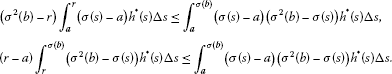And so the result is proved. □

Remark 2.1 The above theorem is also true if we change (H3) by

(${\overline{\mathrm{H}}}_{3}$) For $t\in {\left[a,b\right]}_{T}$ and $x,y\in \mathcal{A}$, there exists $M>0$ such that

${f}_{i}\left(t,x\right)-{f}_{i}\left(t,y\right)\le M\left(x-y\right).$

Remark 2.2 The existence of a lower solution and an upper solution with $0<\alpha \le \beta$ can be obtained through conditions of ${f}_{i}$. For instance, if ${f}_{i}\in {C}_{rd}\left(J\right)$ and ${f}_{i}$ is bounded, the existence holds.

## 3 Existence of a positive solution

Consider the problem

$\left({P}_{0}\right)\phantom{\rule{1em}{0ex}}\left\{\begin{array}{c}-{x}_{i}^{\mathrm{\Delta }\mathrm{\Delta }}\left(t\right)={f}_{i}\left(t,{x}^{\sigma }\left(t\right)\right),\phantom{\rule{1em}{0ex}}t\in {\left({J}^{\kappa }\right)}^{o},\hfill \\ {x}_{i}\left(a\right)={x}_{i}\left({\sigma }^{2}\left(b\right)\right)=0,\phantom{\rule{1em}{0ex}}i=0,\dots ,n.\hfill \end{array}$

We will deduce the existence of a solution to (${P}_{0}$) by supposing that the following hypotheses hold:

(${\stackrel{˜}{\mathrm{H}}}_{1}$) For every $i=1,\dots ,n$, ${f}_{i}:{\left({J}^{\kappa }\right)}^{o}×\mathcal{A}\to \left[0,+\mathrm{\infty }\right)$, where $\mathcal{A}\subset \left(0,+\mathrm{\infty }\right)×{\cdots }^{n}×\left(0,+\mathrm{\infty }\right)$, verifies

1. (i)

For every $x\in \mathcal{A}$, ${f}_{i}\left(\cdot ,x\right)\in {C}_{rd}\left({\left({J}^{\kappa }\right)}^{o}\right)$,

2. (ii)

${f}_{i}\left(t,\cdot \right)$ is continuous on $\mathcal{A}$ uniformly in $t\in {\left({J}^{\kappa }\right)}^{o}$.

(${\stackrel{˜}{\mathrm{H}}}_{2}$) For every $i=1,\dots ,n$, and $j=1,\dots ,n$, there exist constants ${\lambda }_{ij}$, ${\mu }_{ij}$, with $-\mathrm{\infty }<{\lambda }_{ij}<{\mu }_{ij}<1$, ${\lambda }_{ii}<0<{\mu }_{ii}<1$, ${\mu }_{ij}<0$ if $i\ne j$, such that if $0, then

${c}^{{\mu }_{ij}}{f}_{i}\left(t,{x}_{1},\dots ,{x}_{n}\right)\le {f}_{i}\left(t,{x}_{1},\dots ,c{x}_{j},\dots ,{x}_{n}\right)\le {c}^{{\lambda }_{ij}}{f}_{i}\left(t,{x}_{1},\dots ,{x}_{n}\right)$

for each $t\in {\left({J}^{\kappa }\right)}^{o}$ and $x\in \mathcal{A}.$

Remark 3.1 If $c\ge 1$ for every $i=1,\dots ,n$,

${c}^{{\lambda }_{ij}}{f}_{i}\left(t,{x}_{1},\dots ,{x}_{n}\right)\le {f}_{i}\left(t,{x}_{1},\dots ,c{x}_{j},\dots ,{x}_{n}\right)\le {c}^{{\mu }_{ij}}{f}_{i}\left(t,{x}_{1},\dots ,{x}_{n}\right)$

for each $t\in {\left({J}^{\kappa }\right)}^{o}$ and $x\in \mathcal{A}$.

We consider solutions to the problem.

Definition 3.1 A positive solution of type 1 of (${P}_{0}$) is a function $x=\left({x}_{1},\dots ,{x}_{n}\right)$, with ${x}_{i}\in {C}_{rd}^{2}\left({\left(a,b\right)}_{\mathbb{T}}\right)$ for all $i=1,\dots ,n$ such that $x\left(t\right)\in \mathcal{A}$ and ${x}_{i}\left(t\right)>0$ for all $t\in {\left[a,{\sigma }^{2}\left(b\right)\right]}_{\mathbb{T}}$, which satisfies the equalities on (${P}_{0}$) for each $t\in {\left({J}^{\kappa }\right)}^{o}$ and $i=1,\dots ,n$, and the following limits exist and are finite:

$\underset{t\to {a}^{+}}{lim}{x}_{i}^{\mathrm{\Delta }}\left(t\right)\phantom{\rule{1em}{0ex}}\text{and}\phantom{\rule{1em}{0ex}}\underset{t\to \sigma {\left(b\right)}^{-}}{lim}{x}_{i}^{\mathrm{\Delta }}\left(t\right).$

Definition 3.2 We say that $\alpha \in {C}_{rd}^{2}$ is a lower solution of (${P}_{0}$) if for each $i=1,2,\dots ,n$ we have

$\left\{\begin{array}{c}-{\alpha }_{i}^{\mathrm{\Delta }\mathrm{\Delta }}\left(t\right)\le {f}_{i}\left(t,{x}^{\sigma }\left(t\right)\right),\phantom{\rule{1em}{0ex}}t\in {\left({J}^{\kappa }\right)}^{o},\hfill \\ {\alpha }_{i}\left(a\right)={\alpha }_{i}\left({\sigma }^{2}\left(b\right)\right)=0.\hfill \end{array}$

Similarly, $\beta \in {C}_{rd}^{2}$ is called an upper solution of (${P}_{0}$) if for each $i=1,2,\dots ,n$,

$\left\{\begin{array}{c}-{\beta }_{i}^{\mathrm{\Delta }\mathrm{\Delta }}\left(t\right)\ge {f}_{i}\left(t,{x}^{\sigma }\left(t\right)\right),\phantom{\rule{1em}{0ex}}t\in {\left({J}^{\kappa }\right)}^{o},\hfill \\ {\beta }_{i}\left(a\right)={\beta }_{i}\left({\sigma }^{2}\left(b\right)\right)=0.\hfill \end{array}$

Lemma 3.1 Suppose that (${\stackrel{˜}{\mathrm{H}}}_{1}$) and (${\stackrel{˜}{\mathrm{H}}}_{2}$) hold. If x is a positive solution of type 1 of (${P}_{0}$), then for each $i=1,\dots ,n$, there are constants ${I}_{i1}$, ${I}_{i2}$, $0<{I}_{i1}<{I}_{i2}$ such that

${I}_{i1}e\left(t\right)\le {x}_{i}\left(t\right)\le e\left(t\right){I}_{i2},$

where $e\left(t\right)=\frac{\left(t-a\right)\left({\sigma }^{2}\left(b\right)-t\right)}{{\sigma }^{2}\left(b\right)-a}$.

Proof Integrate the equations of (${P}_{0}$) in ${\left({J}^{\kappa }\right)}^{o}$ for $i=1,\dots ,n$

$\begin{array}{rcl}-{\int }_{a}^{\sigma \left(b\right)}{f}_{i}\left(s,{x}^{\sigma }\left(s\right)\right)\mathrm{\Delta }s& =& {\int }_{a}^{\sigma \left(b\right)}{x}_{i}^{\mathrm{\Delta }\mathrm{\Delta }}\left(s\right)\mathrm{\Delta }s\\ =& \underset{t\to \sigma {\left(b\right)}^{-}}{lim}{x}_{i}^{\mathrm{\Delta }}\left(t\right)-\underset{t\to {a}^{+}}{lim}{x}_{i}^{\mathrm{\Delta }}\left(t\right)<+\mathrm{\infty }.\end{array}$

From (2.2), we have

${x}_{i}\left(t\right)={\int }_{a}^{\sigma \left(b\right)}G\left(t,s\right){f}_{i}\left(s,{x}^{\sigma }\left(s\right)\right)\mathrm{\Delta }s\le \frac{\left(t-a\right)\left({\sigma }^{2}\left(b\right)-t\right)}{{\sigma }^{2}\left(b\right)-a}{\int }_{a}^{\sigma \left(b\right)}{f}_{i}\left(s,{x}^{\sigma }\left(s\right)\right)\mathrm{\Delta }s.$

Since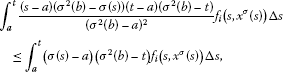and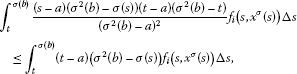we have

$\frac{\left(t-a\right)\left({\sigma }^{2}\left(b\right)-t\right)}{{\left({\sigma }^{2}\left(b\right)-a\right)}^{2}}{\int }_{a}^{\sigma \left(b\right)}\frac{\left(s-a\right)\left({\sigma }^{2}\left(b\right)-\sigma \left(s\right)\right)}{\left({\sigma }^{2}\left(b\right)-a\right)}{f}_{i}\left(s,{x}^{\sigma }\left(s\right)\right)\mathrm{\Delta }s\le {x}_{i}\left(t\right).$

Thus, if we consider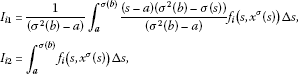it verifies

${I}_{i1}e\left(t\right)\le {x}_{i}\left(t\right)\le e\left(t\right){I}_{i2}.$

□

Lemma 3.2 If α and β are lower and upper solutions of problem (${P}_{0}$) such that $0<\alpha \left(t\right)\le \beta \left(t\right)$ for $t\in {\left({J}^{\kappa }\right)}^{o}$, and (${\stackrel{˜}{\mathrm{H}}}_{1}$) and (H3) or (${\overline{\mathrm{H}}}_{3}$) hold, then problem (${P}_{0}$) has a solution x such that

$\alpha \le x\le \beta .$

If in addition there exists a function $h\left(t\right)=\left({h}_{1}\left(t\right),\dots ,{h}_{n}\left(t\right)\right)$ with ${h}_{i}\in {L}^{1}\left({\left({J}^{\kappa }\right)}^{o}\right)$ such that

$|{f}_{i}\left(t,x\right)|\le {h}_{i}\left(t\right),\phantom{\rule{1em}{0ex}}\mathrm{\forall }t\in {\left({J}^{\kappa }\right)}^{o}\phantom{\rule{1em}{0ex}}\mathit{\text{and}}\phantom{\rule{1em}{0ex}}{\alpha }_{i}\left(t\right)\le {x}_{i}\le {\beta }_{i}\left(t\right),\phantom{\rule{1em}{0ex}}i=1,\dots ,n,$

then the solution x is a positive solution of type 1.

Proof Let us consider ${\left\{{a}_{k}\right\}}_{k\ge 1},{\left\{{b}_{k}\right\}}_{k\ge 1}\subset {\left({J}^{\kappa }\right)}^{o}$ to be two sequences such that ${\left\{{a}_{k}\right\}}_{k\ge 1}\subset {\left(a,\left(a+\sigma \left(b\right)\right)/2\right)}_{\mathbb{T}}$ is strictly decreasing to a if $a=\sigma \left(a\right)$, and ${a}_{k}=a$ for all $k\ge 1$ if $a<\sigma \left(a\right)$, and ${\left\{{b}_{k}\right\}}_{k\ge 1}\subset {\left(\left(a+\sigma \left(b\right)\right)/2,\sigma \left(b\right)\right)}_{\mathbb{T}}$ is strictly increasing to $\sigma \left(b\right)$ if $\rho \left(\sigma \left(b\right)\right)=\sigma \left(b\right)$, ${b}_{k}=\rho \left(\sigma \left(b\right)\right)$ for all $k\ge 1$ if $\rho \left(\sigma \left(b\right)\right)<\sigma \left(b\right)$.

We denote ${D}_{k}:={\left[{a}_{k},{b}_{k}\right]}_{\mathbb{T}}\subset {\left({J}^{\kappa }\right)}^{o}$, $k\ge 1$, and let $\left\{{r}_{i1}^{k}\right\}$, $\left\{{r}_{i2}^{k}\right\}$ be sequences so that

$\begin{array}{r}{\alpha }_{i}\left({a}_{k}\right)\le {r}_{i1}^{k}\le {\beta }_{i}\left({a}_{k}\right),\\ {\alpha }_{i}\left({b}_{k}\right)\le {r}_{i2}^{k}\le {\beta }_{i}\left({k}_{k}\right).\end{array}$

For each $x\in \mathcal{A}$, define

${f}_{ki}^{\ast }\left(\cdot ,x\right):{D}_{k}\to \left[0,\mathrm{\infty }\right)$

for all $k\in \mathbb{N}$, $k\ge 1$ and $i=1,\dots ,n$, as

${f}_{ki}^{\ast }\left(t,x\right)={f}_{i}\left(t,d\left(t,x\right)\right)+\frac{{d}_{i}\left(t,x\right)-{x}_{i}}{1+|{d}_{i}\left(t,x\right)-{x}_{i}|}.$

Consider the problems

$\left({P}_{k}\right)\phantom{\rule{1em}{0ex}}\left\{\begin{array}{c}{x}_{i}^{\mathrm{\Delta }\mathrm{\Delta }}\left(t\right)=-{f}_{ki}^{\ast }\left(t,{x}^{\sigma }\left(t\right)\right),\phantom{\rule{1em}{0ex}}t\in {D}_{k},\hfill \\ {x}_{i}\left({a}_{k}\right)={r}_{i1}^{k},\phantom{\rule{2em}{0ex}}{x}_{i}\left({\sigma }^{2}\left({b}_{k}\right)\right)={r}_{i2}^{k},\phantom{\rule{1em}{0ex}}i=1,\dots ,n.\hfill \end{array}$

Due to the hypothesis ${f}_{i}$, $i=1,\dots ,n$, by Theorem 2.1, we can ensure that there exists a solution $\left({x}_{k1},\dots ,{x}_{kn}\right)$ with ${x}_{ki}\in {C}_{rd}\left[{a}_{k},{\sigma }^{2}\left({b}_{k}\right)\right]$ such that

${\alpha }_{i}\left(t\right)\le {x}_{ki}\left(t\right)\le {\beta }_{i}\left(t\right),$

with $t\in \left[{a}_{k},{b}_{k}\right]$. Since $\left[{a}_{1},{b}_{1}\right]\subset \left[{a}_{k},{b}_{k}\right]$ for $k\in \mathbb{N}$, there exists ${t}_{k}\in \left[{a}_{1},{b}_{1}\right]$ such that

$|{x}_{ki}^{\mathrm{\Delta }}\left({t}_{k}\right)|\le \frac{|{x}_{ki}\left({b}_{1}\right)-{x}_{ki}\left({a}_{1}\right)|}{|{b}_{1}-{a}_{1}|}\le \frac{2\left({\beta }_{i}\left({b}_{1}\right)-{\beta }_{i}\left({a}_{1}\right)\right)}{|{b}_{1}-{a}_{1}|}.$

Thus, we can find a sequence $\left\{{t}_{k}\right\}$ which converges to ${t}_{0}\in \left[{a}_{k},{b}_{k}\right]$ for $k\in \mathbb{N}$, satisfying

${x}_{ki}\left({t}_{k}\right)\to {x}_{0i}\in \left[{\alpha }_{i}\left({t}_{0}\right),{\beta }_{i}\left({t}_{0}\right)\right],$

and

${x}_{ki}^{\mathrm{\Delta }}\left({t}_{k}\right)\to {x}_{0i}^{\mathrm{\Delta }}$

for $i=1,\dots ,n$ when $k\to \mathrm{\infty }$.

We note that ${x}_{ki}$ is the solution of

${y}_{i}^{\mathrm{\Delta }\mathrm{\Delta }}\left(t\right)=-{f}_{i}\left(t,{y}^{\sigma }\left(t\right)\right),$

with ${y}_{i}\left({t}_{k}\right)={x}_{ki}\left({t}_{k}\right)$ and ${y}_{i}^{\mathrm{\Delta }}\left({t}_{k}\right)={x}_{ki}^{\mathrm{\Delta }}\left({t}_{k}\right)$.

Hence, due to an adaptation of Theorem 3.2 in  and by existence theorems, we can find a solution of the problem

${x}_{i}^{\mathrm{\Delta }\mathrm{\Delta }}\left(t\right)=-{f}_{i}\left(t,{x}^{\sigma }\left(t\right)\right),\phantom{\rule{2em}{0ex}}{x}_{i}\left({t}_{0}\right)={x}_{0i},\phantom{\rule{2em}{0ex}}{x}_{i}^{\mathrm{\Delta }}\left({t}_{0}\right)={x}_{0i}^{\mathrm{\Delta }},\phantom{\rule{1em}{0ex}}i=1,\dots ,n.$

This solution is defined in a maximal interval W, and we can find at least one sequence $\left\{{x}_{k}\left(t\right)\right\}$ that converges uniformly to $x\left(t\right)$ in the compact subintervals of W.

On the other hand, ${\bigcup }_{k=1}^{\mathrm{\infty }}\left[{a}_{k},{b}_{k}\right]={\left({J}^{\kappa }\right)}^{o}$ and ${\alpha }_{i}\left(t\right)\le {x}_{ki}\left(t\right)\le {\beta }_{i}\left(t\right)$ for $t\in \left[{a}_{k},{b}_{k}\right]$, then x is defined in ${\left({J}^{\kappa }\right)}^{o}$ and $\alpha \left(t\right)\le x\left(t\right)\le \beta \left(t\right)$ for all $t\in {\left({J}^{\kappa }\right)}^{o}$. From the conditions on α and β on the boundary, it follows that

$x\left(a\right)=x\left({\sigma }^{2}\left(b\right)\right)=0,$

so that x is a solution of problem (${P}_{0}$).

Suppose there exists a function $h\left(t\right)=\left({h}_{1}\left(t\right),\dots ,{h}_{n}\left(t\right)\right)$ with ${h}_{i}\in {L}^{1}\left({\left({J}^{\kappa }\right)}^{o}\right)$ such that

$|{f}_{i}\left(t,x\right)|\le {h}_{i}\left(t\right),\phantom{\rule{1em}{0ex}}\mathrm{\forall }t\in {\left({J}^{\kappa }\right)}^{o}\phantom{\rule{1em}{0ex}}\text{and}\phantom{\rule{1em}{0ex}}{\alpha }_{i}\left(t\right)\le {x}_{i}\le {\beta }_{i}\left(t\right),\phantom{\rule{1em}{0ex}}i=1,\dots ,n,$

then we can assume that $|{x}_{i}^{\mathrm{\Delta }\mathrm{\Delta }}\left(t\right)|\le {h}_{i}\left(t\right)$, $i=1,\dots ,n$, which implies that ${x}_{i}^{\mathrm{\Delta }\mathrm{\Delta }}$ is absolutely integrable on ${\left[a,\sigma \left(b\right)\right]}_{\mathbb{T}}$ and ${x}_{i}^{\mathrm{\Delta }}\in C\left[a,\sigma \left(b\right)\right]$, $i=1,\dots ,n$, so x is a positive solution of type 1. □

Theorem 3.1 Suppose that (${\stackrel{˜}{\mathrm{H}}}_{1}$), (${\stackrel{˜}{\mathrm{H}}}_{2}$) and (H3) or ($\overline{{\mathrm{H}}_{3}}$) hold. There exists a positive solution of type 1 if and only if the following conditions hold:

$0<{\int }_{a}^{\sigma \left(b\right)}{f}_{i}\left(s,{E}^{\sigma }\left(s\right)\right)\mathrm{\Delta }s<\mathrm{\infty },$

for all $i=1,\dots ,n$, where $E\left(t\right)=\left(e\left(t\right),\dots ,e\left(t\right)\right)$.

Proof Necessity. Suppose that there exists $x=\left({x}_{1},\dots ,{x}_{n}\right)$ positive solutions of type 1 of (${P}_{0}$). By Lemma 3.1, there are constants ${I}_{i1}$, ${I}_{i2}$, $0<{I}_{i1}<{I}_{i2}$ for each $i=1,\dots ,n$ such that

${I}_{i1}e\left(t\right)\le {x}_{i}\left(t\right)\le e\left(t\right){I}_{i2}.$

Let $K>0$ such that $K{I}_{i2}\le 1$, $\frac{1}{K}\ge 1$, $i=1,\dots ,n$. By (${\stackrel{˜}{\mathrm{H}}}_{2}$) and the above inequality, it follows that

$\begin{array}{rcl}{f}_{i}\left(t,{x}^{\sigma }\left(t\right)\right)& \ge & {\left(\frac{1}{K}\right)}^{{\lambda }_{ii}}{f}_{i}\left(t,{x}_{1}^{\sigma }\left(t\right),\dots ,\frac{K{x}_{i}^{\sigma }\left(t\right)}{{e}^{\sigma }\left(t\right)}{e}^{\sigma }\left(t\right),\dots ,{x}_{n}^{\sigma }\left(t\right)\right)\\ \ge & {K}^{{\mu }_{ii}-{\lambda }_{ii}}{\left(\frac{{x}_{i}^{\sigma }\left(t\right)}{{e}^{\sigma }\left(t\right)}\right)}^{{\mu }_{ii}}{f}_{i}\left(t,{x}_{1}^{\sigma }\left(t\right),\dots ,{e}^{\sigma }\left(t\right),\dots ,{x}_{n}^{\sigma }\left(t\right)\right)\\ \ge & {K}^{{\mu }_{ij}-{\lambda }_{ii}}{I}_{i1}^{{\mu }_{ii}}{f}_{i}\left(t,{x}_{1}^{\sigma }\left(t\right),\dots ,{e}^{\sigma }\left(t\right),\dots ,{x}_{n}^{\sigma }\left(t\right)\right)\\ \ge & {K}^{{\sum }_{j=1}^{n}\left({\mu }_{ij}-{\lambda }_{ij}\right)}{I}_{i1}^{{\mu }_{ii}}\prod _{j=1,j\ne i}^{n}{I}_{j2}^{{\mu }_{ij}}{f}_{i}\left(t,{E}^{\sigma }\left(t\right)\right),\end{array}$

hence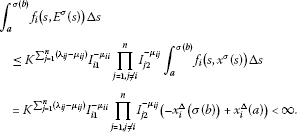Sufficiency. Suppose that there exists a constant $C\ge 1$ such that $C{I}_{i1}\ge 1$ and ${I}_{i2}\le C$.

We consider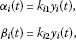with

${y}_{i}\left(t\right)={\int }_{a}^{\sigma \left(b\right)}G\left(t,s\right){f}_{i}\left(s,{E}^{\sigma }\left(s\right)\right)\mathrm{\Delta }s,$

where $G\left(t,s\right)$ is Green’s function (2.2) and ${k}_{i1}$ and ${k}_{i2}$ are determined below.

Note that ${y}_{i}$ satisfies

• ${y}_{i},{y}_{i}^{\mathrm{\Delta }}\in C\left({\left[a,{\sigma }^{2}\left(b\right)\right]}_{\mathbb{T}}\right)$,

• ${y}_{i}^{\mathrm{\Delta }\mathrm{\Delta }}\in {C}_{rd}\left({\left(a,{\sigma }^{2}\left(b\right)\right)}_{\mathbb{T}}\right)$.

We have

$e\left(t\right){I}_{i1}\le {y}_{i}\left(t\right)\le e\left(t\right){I}_{i2},$

where

${I}_{i1}=\frac{1}{{\sigma }^{2}\left(b\right)-a}{\int }_{a}^{\sigma \left(b\right)}\frac{\left(s-a\right)\left({\sigma }^{2}\left(b\right)-\sigma \left(s\right)\right)}{{\sigma }^{2}\left(b\right)-a}{f}_{i}\left(s,{e}^{\sigma }\left(s\right)\right)\mathrm{\Delta }s,$

and

${I}_{i2}={\int }_{a}^{\sigma \left(b\right)}{f}_{i}\left(s,{e}^{\sigma }\left(s\right)\right)\mathrm{\Delta }s,$

Consider now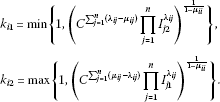Given that ${e}^{\sigma }\left(t\right){I}_{i1}\le {y}_{i}^{\sigma }\left(t\right)$ and $C{I}_{i1}\ge 1$, then ${e}^{\sigma }\left(t\right)\le C{y}_{i}^{\sigma }\left(t\right)$. Since

${f}_{i}\left(t,{\alpha }_{1}^{\sigma }\left(t\right),\dots ,{\alpha }_{n}^{\sigma }\left(t\right)\right)={f}_{i}\left(t,\frac{{k}_{11}}{C}\frac{C{y}_{1}^{\sigma }\left(t\right)}{{e}^{\sigma }\left(t\right)}{e}^{\sigma }\left(t\right),\dots ,\frac{{k}_{n1}}{C}\frac{C{y}_{n}^{\sigma }\left(t\right)}{{e}^{\sigma }\left(t\right)}{e}^{\sigma }\left(t\right)\right),$

we have that

$\begin{array}{rcl}{f}_{i}\left(t,{\alpha }^{\sigma }\left(t\right)\right)& \ge & \prod _{j=1}^{n}{\left(\frac{{k}_{j1}}{C}\right)}^{{\mu }_{ij}}{\left(\frac{C{y}_{j}^{\sigma }\left(t\right)}{{e}^{\sigma }\left(t\right)}\right)}^{{\lambda }_{ij}}{f}_{i}\left(t,{E}^{\sigma }\left(t\right)\right)\\ \ge & \prod _{j=1}^{n}{k}_{j1}^{{\mu }_{ij}}{C}^{{\lambda }_{ij}-\mu ij}{I}_{j2}^{{\lambda }_{ij}}{f}_{i}\left(t,{E}^{\sigma }\left(t\right)\right),\end{array}$

and

$\prod _{j=1}^{n}{k}_{j1}^{{\mu }_{ij}}{C}^{{\lambda }_{ij}-\mu ij}{I}_{j2}^{{\lambda }_{ij}}\ge {k}_{i1}^{1-{\mu }_{ii}}\prod _{j=1}^{n}{k}_{j1}^{{\mu }_{ij}}\ge {k}_{i1}\prod _{j=1,i\ne j}^{n}{k}_{j1}^{{\mu }_{ij}}\ge {k}_{i1},$

which implies that

${f}_{i}\left(t,{\alpha }^{\sigma }\left(t\right)\right)\ge {k}_{i1}{f}_{i}\left(t,{E}^{\sigma }\left(t\right)\right)=-{\alpha }_{i}^{\mathrm{\Delta }\mathrm{\Delta }}\left(t\right).$

In a similar way,

$\begin{array}{rcl}{f}_{i}\left(t,{\beta }^{\sigma }\left(t\right)\right)& \le & \prod _{j=1}^{n}{\left({k}_{j2}C\right)}^{{\mu }_{ij}}{\left(\frac{{y}_{j}^{\sigma }\left(t\right)}{C{e}^{\sigma }\left(t\right)}\right)}^{{\lambda }_{ij}}{f}_{i}\left(t,{E}^{\sigma }\left(t\right)\right)\\ \le & \prod _{j=1}^{n}{k}_{j2}^{{\mu }_{ij}}{C}^{{\mu }_{ij}-\lambda ij}{I}_{j1}^{{\lambda }_{ij}}{f}_{i}\left(t,{E}^{\sigma }\left(t\right)\right)\le {k}_{i2}{f}_{i}\left(t,{E}^{\sigma }\left(t\right)\right)=-{\beta }_{i}^{\mathrm{\Delta }\mathrm{\Delta }}\left(t\right).\end{array}$

Thus, there is a lower solution α and an upper solution β of problem (${P}_{0}$) that satisfy $0<{\alpha }_{i}\left(t\right)\le {\beta }_{i}\left(t\right)$ for $t\in {\left({J}^{\kappa }\right)}^{o}$, $i=1,\dots ,n$, ${\alpha }_{i}\left(a\right)={\beta }_{i}\left(a\right)={\alpha }_{i}\left({\sigma }^{2}\left(b\right)\right)={\beta }_{i}\left({\sigma }^{2}\left(b\right)\right)=0$. Applying Lemma 3.2, problem (${P}_{0}$) has a solution x such that $\alpha \le x\le \beta$. Note that for $t\in {\left({J}^{\kappa }\right)}^{o}$ and $\alpha \le x\le \beta$,

$\begin{array}{rcl}0& \le & {f}_{i}\left(t,{x}_{i}^{\sigma }\left(t\right)\right)={f}_{i}\left(t,\left(\frac{{k}_{11}}{C}\right)\left(\frac{C{x}_{1}^{\sigma }\left(t\right)}{{k}_{11}{e}^{\sigma }\left(t\right)}\right){e}^{\sigma }\left(t\right),\dots ,\left(\frac{{k}_{n1}}{C}\right)\left(\frac{C{x}_{n}^{\sigma }\left(t\right)}{{k}_{n1}{e}^{\sigma }\left(t\right)}\right){e}^{\sigma }\left(t\right)\right)\\ \le & {\left(\frac{{k}_{11}}{C}\right)}^{{\lambda }_{i1}}{\left(\frac{C{x}_{1}^{\sigma }\left(t\right)}{{k}_{11}{e}^{\sigma }\left(t\right)}\right)}^{{\mu }_{i1}}\cdots {\left(\frac{{k}_{n1}}{C}\right)}^{{\lambda }_{in}}{\left(\frac{C{x}_{n}^{\sigma }\left(t\right)}{{k}_{n1}{e}^{\sigma }\left(t\right)}\right)}^{{\mu }_{in}}{f}_{i}\left(t,{E}^{\sigma }\left(t\right)\right)\le {h}_{i}\left(t\right),\end{array}$

with ${h}_{i}\left(t\right)={K}_{i}{f}_{i}\left(t,{E}^{\sigma }\left(t\right)\right)$ and

${K}_{i}=\prod _{j=1}^{n}{\left(\frac{{k}_{j1}}{C}\right)}^{{\lambda }_{ij}-{\mu }_{ij}}\prod _{j=1}^{n}\underset{k=1,\dots ,n}{max}\left\{{\left({I}_{k1}\right)}^{{\mu }_{ij}},{\left({I}_{k2}\right)}^{{\mu }_{ij}}\right\}.$

Due to the hypothesis, we can then ensure that

${\int }_{a}^{\sigma \left(b\right)}{h}_{i}\left(s\right)\mathrm{\Delta }s<\mathrm{\infty },$

for $i=1,\dots ,n$, which implies the existence of a positive solution of type 1 of problem (${P}_{0}$) such that $0<\alpha \le x\le \beta$. □

Theorem 3.2 If there exists a positive solution of the problem and ${\sigma }^{2}\left(b\right)>0$, then the following conditions hold:

$0<{\int }_{a}^{\sigma \left(b\right)}\left(\sigma \left(s\right)-a\right)\left(\sigma \left(b\right)-\sigma \left(s\right)\right){f}_{i}\left(s,\left[{\sigma }^{2}\left(b\right)\right]\right)\mathrm{\Delta }s<\mathrm{\infty }$

for all $i=1,\dots ,n$, with $\left[{\sigma }^{2}\left(b\right)\right]=\left({\sigma }^{2}\left(b\right),\dots ,{\sigma }^{2}\left(b\right)\right)$.

Proof Fix $i=1,\dots ,n$, let us consider ${C}_{0}>0$, ${C}_{0}^{i}$ to be two constants such that $\frac{{C}_{0}{x}_{j}^{\sigma }\left(t\right)}{{\sigma }^{2}\left(b\right)}\le 1$ if $j=1,\dots ,n$ and $j\ne i$, $\frac{1}{{C}_{0}}\ge 1$, $\frac{{C}_{0}^{i}{x}_{i}^{\sigma }\left(t\right)}{{\sigma }^{2}\left(b\right)}\ge 1$ and ${C}_{0}^{i}\ge 1$.

We have

$\begin{array}{rcl}{f}_{i}\left(t,{x}^{\sigma }\left(t\right)\right)& \ge & \left(\prod _{j=1,j\ne i}^{n}{\left(\frac{1}{{C}_{0}}\right)}^{\lambda ij}{\left(\frac{{C}_{0}{x}_{j}^{\sigma }\left(t\right)}{{\sigma }^{2}\left(b\right)}\right)}^{\mu ij}\right){f}_{i}\left(t,{\sigma }^{2}\left(b\right),\dots ,{x}_{i}^{\sigma }\left(t\right),\dots ,{\sigma }^{2}\left(b\right)\right)\\ \ge & \left(\prod _{j=1,j\ne i}^{n}{\left(\frac{1}{{C}_{0}}\right)}^{\lambda ij}{\left(\frac{{C}_{0}{x}_{j}^{\sigma }\left(t\right)}{{\sigma }^{2}\left(b\right)}\right)}^{\mu ij}\right){\left(\frac{1}{{C}_{0}^{i}}\right)}^{\mu ii}{\left(\frac{{C}_{0}^{i}{x}_{i}^{\sigma }\left(t\right)}{{\sigma }^{2}\left(b\right)}\right)}^{\lambda ii}{f}_{i}\left(t,\left[{\sigma }^{2}\left(b\right)\right]\right).\end{array}$

Hence,

${f}_{i}\left(t,\left[{\sigma }^{2}\left(b\right)\right]\right)\le M{f}_{i}\left(t,{x}^{\sigma }\left(t\right)\right),$

where

$M=\underset{t\in {\left[a,\sigma \left(b\right)\right]}_{\mathbb{T}}}{sup}\left(\prod _{j=1,j\ne i}^{n}{C}_{0}^{\lambda ij-\mu ij}{\left(\frac{{x}_{j}^{\sigma }\left(t\right)}{{\sigma }^{2}\left(b\right)}\right)}^{-\mu ij}\right){\left({C}_{0}^{i}\right)}^{\mu ii}{\left(\frac{{C}_{0}^{i}{x}_{i}^{\sigma }\left(t\right)}{{\sigma }^{2}\left(b\right)}\right)}^{-\lambda ii}.$

Let ${t}_{i}\in {\left(a,\sigma \left(b\right)\right)}_{\mathbb{T}}$,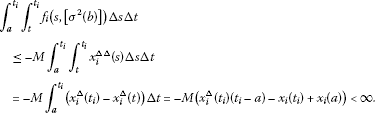Integrating by parts, we have

${\int }_{a}^{{t}_{i}}{\int }_{t}^{{t}_{i}}{f}_{i}\left(s,\left[{\sigma }^{2}\left(b\right)\right]\right)\mathrm{\Delta }s\mathrm{\Delta }t={\int }_{a}^{{t}_{i}}\left(\sigma \left(t\right)-a\right){f}_{i}\left(t,{x}_{{\sigma }^{2}\left(b\right)}\right)\mathrm{\Delta }t<\mathrm{\infty }.$

In a similar way,

$\begin{array}{rcl}{\int }_{{t}_{i}}^{\sigma \left(b\right)}{\int }_{{t}_{i}}^{t}{f}_{i}\left(s,\left[{\sigma }^{2}\left(b\right)\right]\right)\mathrm{\Delta }s\mathrm{\Delta }t& \le & -M{\int }_{{t}_{i}}^{\sigma \left(b\right)}{\int }_{{t}_{i}}^{t}{x}_{i}^{\mathrm{\Delta }\mathrm{\Delta }}\left(s\right)\mathrm{\Delta }s\mathrm{\Delta }t\\ =& -M{\int }_{{t}_{i}}^{\sigma \left(b\right)}\left({x}_{i}^{\mathrm{\Delta }}\left(t\right)-{x}_{i}^{\mathrm{\Delta }}\left({t}_{i}\right)\right)\mathrm{\Delta }t\\ =& -M\left({x}_{i}\left(\sigma \left(b\right)\right)-{x}_{i}\left({t}_{i}\right)-{x}_{i}^{\mathrm{\Delta }}\left({t}_{i}\right)\left(\sigma \left(b\right)-{t}_{i}\right)\right)<\mathrm{\infty },\end{array}$

and integrating by parts,

${\int }_{{t}_{i}}^{\sigma \left(b\right)}{\int }_{{t}_{i}}^{t}{f}_{i}\left(s,\left[{\sigma }^{2}\left(b\right)\right]\right)\mathrm{\Delta }s\mathrm{\Delta }t={\int }_{{t}_{i}}^{\sigma \left(b\right)}\left(\sigma \left(b\right)-\sigma \left(t\right)\right){f}_{i}\left(t,\left[{\sigma }^{2}\left(b\right)\right]\right)\mathrm{\Delta }t<\mathrm{\infty }.$

Then we conclude that

$0<{\int }_{a}^{\sigma \left(b\right)}\left(\sigma \left(t\right)-a\right)\left(\sigma \left(b\right)-\sigma \left(t\right)\right){f}_{i}\left(t,\left[{\sigma }^{2}\left(b\right)\right]\right)\mathrm{\Delta }t<\mathrm{\infty }.$

□

Theorem 3.3 If the following conditions hold:

$0<{\int }_{a}^{\sigma \left(b\right)}\left(\sigma \left(s\right)-a\right)\left(\sigma \left(b\right)-\sigma \left(s\right)\right){f}_{i}\left(s,\left[{\sigma }^{2}\left(b\right)\right]\right)\mathrm{\Delta }s<\mathrm{\infty }$

for all $i=1,\dots ,n$ and ${\sigma }^{2}\left(b\right)>0$, then there exists a lower solution to problem (P).

Proof Consider the function

${g}_{i}\left(t\right)={\int }_{a}^{\sigma \left(b\right)}G\left(t,s\right)\frac{\left(\sigma \left(s\right)-a\right)\left({\sigma }^{2}\left(b\right)-\sigma \left(s\right)\right)}{{\left({\sigma }^{2}\left(b\right)-a\right)}^{2}}{f}_{i}\left(s,\left[{\sigma }^{2}\left(b\right)\right]\right)\mathrm{\Delta }s.$

Let us see that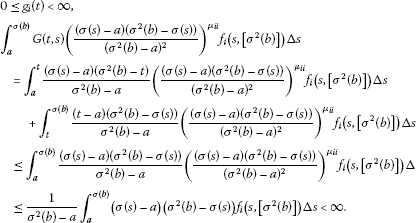Furthermore, ${g}_{i}\left(a\right)={g}_{i}\left({\sigma }^{2}\left(b\right)\right)=0$.

If we consider

${L}_{i1}=\frac{1}{{\sigma }^{2}\left(b\right)-a}{\int }_{a}^{\sigma \left(b\right)}\left(\frac{\left(\sigma \left(s\right)-a\right){\left({\sigma }^{2}\left(b\right)-\sigma \left(s\right)\right)}^{1+{\mu }_{ii}}}{{\left({\sigma }^{2}\left(b\right)-a\right)}^{2{\mu }_{ii}}}\right){f}_{i}\left(s,\left[{\sigma }^{2}\left(b\right)\right]\right)\mathrm{\Delta }s.$

Then

${g}_{i}\left(t\right)\le {L}_{i1}.$

On the other hand,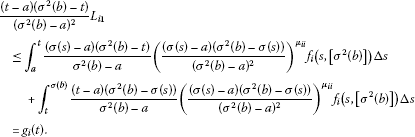Let ${\alpha }_{i}\left(t\right)={k}_{i1}{g}_{i}\left(t\right)$, where

${k}_{i1}=min{\left\{1,\prod _{j=1}^{n}{L}_{j1}^{{\mu }_{ij}}{\left(\frac{1}{{\sigma }^{2}\left(b\right)}\right)}^{{\lambda }_{ij}}{C}_{2}^{\mu ij-\lambda ij}\right\}}^{\frac{1}{1-{\mu }_{ii}}},$

with ${C}_{2}$ being a constant such that ${\sigma }^{2}\left(b\right){C}_{2}{L}_{i1}\le 1$ and $\frac{1}{{C}_{2}{\sigma }^{2}\left(b\right)}\ge 1$.

Thus, if we note that $0<{\mu }_{ii}<1$ and ${\mu }_{ij}<0$ if $i\ne j$, we obtain

${k}_{i1}{L}_{i1}{\left[\frac{\left(t-a\right)\left({\sigma }^{2}\left(b\right)-t\right)}{{\left({\sigma }^{2}\left(b\right)-a\right)}^{2}}\right]}^{\mu ii}\le {\alpha }_{i}{\left(t\right)}^{{\mu }_{ii}},$

and

${\alpha }_{j}{\left(t\right)}^{{\mu }_{ij}}\ge {L}_{j1}^{{\mu }_{ij}}.$

Hence,

$\begin{array}{rcl}{f}_{i}\left(t,{\alpha }^{\sigma }\left(t\right)\right)& \ge & \prod _{j=1}^{n}{\left(\frac{1}{{C}_{2}{\sigma }^{2}\left(b\right)}\right)}^{{\lambda }_{ij}}{\left({C}_{2}{\alpha }_{i}^{\sigma }\left(t\right)\right)}^{{\mu }_{ij}}{f}_{i}\left(t,\left[{\sigma }^{2}\left(b\right)\right]\right)\\ =& \prod _{j=1}^{n}\frac{1}{{\sigma }^{2}{\left(b\right)}^{{\lambda }_{ij}}}{C}_{2}^{{\mu }_{ij}-{\lambda }_{ij}}{\left({\alpha }_{j}^{\sigma }\left(t\right)\right)}^{{\mu }_{ij}}{f}_{i}\left(t,\left[{\sigma }^{2}\left(b\right)\right]\right)\\ \ge & \prod _{j=1,j\ne i}^{n}\frac{1}{{\sigma }^{2}{\left(b\right)}^{{\lambda }_{ii}+{\lambda }_{ij}}}{C}_{2}^{{\mu }_{ij}-{\lambda }_{ij}}{C}_{2}^{{\mu }_{ii}-{\lambda }_{ii}}{L}_{j1}^{{\mu }_{ij}}{\left({\alpha }_{i}^{\sigma }\left(t\right)\right)}^{{\mu }_{ii}}{f}_{i}\left(t,\left[{\sigma }^{2}\left(b\right)\right]\right)\\ \ge & {k}_{i1}{\left(\frac{\left({\sigma }^{2}\left(b\right)-\sigma \left(t\right)\right)\left(\sigma \left(t\right)-a\right)}{{\left({\sigma }^{2}\left(b\right)-a\right)}^{2}}\right)}^{{\mu }_{ii}}{f}_{i}\left(t,\left[{\sigma }^{2}\left(b\right)\right]\right)=-{\alpha }_{i}^{\mathrm{\Delta }\mathrm{\Delta }}\left(t\right).\end{array}$

This implies that α is a lower solution of problem (${P}_{0}$). □

Theorem 3.4 Suppose that the conditions of the above theorem are satisfied and consider α the lower solution of problem (${P}_{0}$) provided. If there exists β, an upper solution of (${P}_{0}$), with $0<\alpha \le \beta$ and (${\stackrel{˜}{\mathrm{H}}}_{1}$) and (H3) or (${\overline{\mathrm{H}}}_{3}$) hold, then there exists x a positive solution of (${P}_{0}$).

Proof The demonstration of this fact is immediate taking into account the construction of the lower solution α obtained in the previous theorem, the existence of the upper solution β with $0<\alpha \le \beta$ and the implementation of Lemma 3.2. □

### 3.1 Particular cases

Let us briefly consider the following examples.

1. 1

If ${\left[a,{\sigma }^{2}\left(b\right)\right]}_{\mathbb{T}}$ is bounded and consists of only isolated points such as in the case $\mathbb{T}=h\mathbb{Z}$, then the conditions of Theorems 3.1 and 3.2 are fulfilled. This follows from the fact

${\int }_{a}^{{\sigma }^{2}\left(b\right)}f\left(t\right)\mathrm{\Delta }t=\sum _{t\in \left[a,{\sigma }^{2}\left(b\right)\right)}\left(\sigma \left(t\right)-t\right)f\left(t\right).$
2. 2

Let $q>1$ be fixed, the quantum time scale $\overline{{q}^{\mathbb{Z}}}$ is defined as

$\overline{{q}^{\mathbb{Z}}}=\left\{{q}^{k}:k\in \mathbb{Z}\right\}\cup \left\{0\right\},$

which appears throughout the mathematical physics literature, where the dynamical systems of interest are the q-difference equations.

Since the only non-isolated point is 0, the interesting case is the one in which the interval contains this point. We consider $a=0$ and $\sigma \left(b\right)=1$.

Taking into account the fact that

${\int }_{0}^{1}f\left(t\right)\mathrm{\Delta }t=\sum _{k=1}^{\mathrm{\infty }}\frac{q-1}{{q}^{k}}f\left({q}^{-k}\right),$

we have

${\int }_{0}^{1}{f}_{i}\left(s,{E}^{\sigma }\left(s\right)\right)\mathrm{\Delta }s=\sum _{k=1}^{\mathrm{\infty }}\frac{q-1}{{q}^{k}}{f}_{i}\left({q}^{-k},{E}^{\sigma }\left({q}^{-k}\right)\right),$

with ${E}^{\sigma }\left({q}^{-k}\right)=\left({q}^{-k+1}\left(1-{q}^{-k}\right),\dots ,{q}^{-k+1}\left(1-{q}^{-k}\right)\right)$. Hence, the convergence of this series is the necessary and sufficient condition in Theorem 3.1.

Analogously, the condition in Theorem 3.2 can be rewritten as

$0<{\int }_{0}^{1}{q}^{-2k+1}\left(q-1\right)\left(1-{q}^{-k+1}\right){f}_{i}\left({q}^{-k},\left(q,\dots ,q\right)\right)\mathrm{\Delta }s<\mathrm{\infty }.$

## References

1. Hilger, S: Ein Maßkettenkalkül mit Anwendung auf Zentrumsmannigfaltigkeiten. Universität Würzburg (1988) (in German)

2. Jain B, Sheng AD: An exploration of the approximation of derivative functions via finite differences. Rose-Hulman Undergraduate Math J. 2007, 8: 1–19.

3. Atici FM, Biles DC, Lebedinsky A: An application of time scales to economics. Math. Comput. Model. 2006, 43: 718–726. 10.1016/j.mcm.2005.08.014

4. Wong JSW: On the generalized Emden-Fowler equation. SIAM Rev. 1975, 17: 339–360. 10.1137/1017036

5. Agarwal RP, O’Regan D, Lakshmikantham V, Leela S: An upper and lower solution theory for singular Emden-Fowler equations. Nonlinear Anal., Real World Appl. 2002, 3: 275–291. 10.1016/S1468-1218(01)00029-3

6. Habets P, Zanolin F: Upper and lower solutions for a generalized Emden-Fowler equations. J. Math. Anal. Appl. 1994, 181: 684–700. 10.1006/jmaa.1994.1052

7. Zhang Y: Positive solutions of singular sublinear Emden-Fowler boundary value problems. J. Math. Anal. Appl. 1994, 185: 215–222. 10.1006/jmaa.1994.1243

8. Wei Z: Positive solution of singular Dirichlet boundary value problems for second order differential equation system. J. Math. Anal. Appl. 2007, 328: 1255–1267. 10.1016/j.jmaa.2006.06.053

9. Agarwal RP, Bohner M, Grace SR, O’Regan D: Discrete Oscillation Theory. Hindawi Publishing Corporation, New York; 2005.

10. Akin-Bohner E, Hoffacker J: Oscillation properties of an Emden-Fowler type equation on discrete time scales. J. Differ. Equ. Appl. 2003, 9: 603–612. 10.1080/1023619021000053575

11. Gómez A, Otero-Espinar V: Existence and uniqueness of positive solution for singular BVPs on time scales. Adv. Differ. Equ. 2009., 2009: Article ID 728484

12. Khan RA, Nieto JJ, Otero-Espinar V: Existence and approximation of solution of three-point boundary value problems on time scale. J. Differ. Equ. Appl. 2008, 14: 723–736. 10.1080/10236190701840906

13. Zhao J, Lian H, Ge W: Existence of positive solutions for nonlinear m-point boundary value problems on time scales. Bound. Value Probl. 2012., 2012: Article ID 4

14. Agarwal RP, Otero–Espinar V, Perera K, Vivero DR: Multiple positive solutions in the sense of distributions of singular BVPs on time scales and a application to Emden-Fowler equations. Adv. Differ. Equ. 2008., 2008: Article ID 796851

15. Han Z, Sun S, Shi B: Oscillation criteria for a class of second-order Emden-Fowler delay dynamic equations on time scales. J. Math. Anal. Appl. 2007, 334: 847–858. 10.1016/j.jmaa.2007.01.004

16. Bohner M, Erbe L, Peterson A: Oscillation for nonlinear second order dynamic equations on a time scale. J. Math. Anal. Appl. 2005, 301: 491–507. 10.1016/j.jmaa.2004.07.038

17. Bohner M, Peterson A: Dynamic Equations on Time Scales: An Introduction with Applications. Birkhauser, Boston; 2001.

18. Hartman P: Ordinary Differential Equations. Birkhauser, Boston; 1982.

## Acknowledgements

The authors are grateful to the referees for their valuable suggestions that led to the improvement of the original manuscript.The research of VOE has been partially supported by Ministerio de Educación y Ciencia (Spain) and FEDER, Project MTM2010-15314. The research of AL has been supported by FSE y de la DX de Ordenación y Calidad del Sistema Universitario de Galicia, de la Conselleria de Educación y Ordenación Universitaria-Xunta de Galicia.

## Author information

Authors

### Corresponding author

Correspondence to Victoria Otero-Espinar.

### Competing interests

The authors declare that they have no competing interests.

### Authors’ contributions

All authors contributed equally in this article. They read and approved the final manuscript.

## Rights and permissions

Open Access This article is distributed under the terms of the Creative Commons Attribution 2.0 International License ( https://creativecommons.org/licenses/by/2.0 ), which permits unrestricted use, distribution, and reproduction in any medium, provided the original work is properly cited.

Reprints and Permissions

Lago, A., Otero-Espinar, V. & Pernas, T. Positive solution of singular BVPs for a system of dynamic equations on time scales. Adv Differ Equ 2012, 185 (2012). https://doi.org/10.1186/1687-1847-2012-185